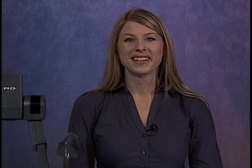PLAY PREVIEW

### Segments in this Video

#### Correlation and Regression Lecture Outline(01:26)

FREE PREVIEW

In this video lecture, Professor Naomi Lowe will discuss logic, assumptions of correlation, calculations of Pearson's r, and regression lines.

#### Logic Behind Correlation and Regression (10:46)

Correlations examine the relationship between two continuous variables. The relationships between the variables are represented on scatter plots. R values range from -1 to 1.

#### Correlation and Causation (04:17)

A positive relationship between two variables does not mean they have a causal effect. Confounding variables can influence both variables at the same time.

#### Assumptions for Correlation (05:31)

Linearity, normality of residuals, and homoscedasticity are three assumptions made when using correlation for statistical analysis.

#### Calculating r(05:04)

Lowe introduces the formula for calculating r. To test significance, an r distribution is converted to t. r is also an effect size

#### Regression (03:05)

Regression makes predictions based on one variable and a regression line of best fit equation.

For additional digital leasing and purchase options contact a media consultant at 800-257-5126
(press option 3) or sales@films.com.

# Correlation and Regression: Lecture 10

Part of the Series : Statistics With Naomi Lowe
 DVD (Chaptered) Price: \$129.95 DVD + 3-Year Streaming Price: \$194.93 3-Year Streaming Price: \$129.95

Share

### Description

In this video lecture, Professor Naomi Lowe discusses logic, assumptions of correlation, calculations of Pearson's r, and regression lines.

Length: 31 minutes

Item#: BVL150110

ISBN: 978-1-64347-562-2Closed Captioned